## Electric field

7-7-99

### Electric field

To help visualize how a charge, or a collection of charges, influences the region around it, the concept of an electric field is used. The electric field E is analogous to g, which we called the acceleration due to gravity but which is really the gravitational field. Everything we learned about gravity, and how masses respond to gravitational forces, can help us understand how electric charges respond to electric forces.

The electric field a distance r away from a point charge Q is given by:

Electric field from a point charge : E = k Q / r2

The electric field from a positive charge points away from the charge; the electric field from a negative charge points toward the charge. Like the electric force, the electric field E is a vector. If the electric field at a particular point is known, the force a charge q experiences when it is placed at that point is given by :

F = qE

If q is positive, the force is in the same direction as the field; if q is negative, the force is in the opposite direction as the field.

### Learning from gravity

Right now you are experiencing a uniform gravitational field: it has a magnitude of 9.8 m/s2 and points straight down. If you threw a mass through the air, you know it would follow a parabolic path because of gravity. You could determine when and where the object would land by doing a projectile motion analysis, separating everything into x and y components. The horizontal acceleration is zero, and the vertical acceleration is g. We know this because a free-body diagram shows only mg, acting vertically, and applying Newton's second law tells us that mg = ma, so a = g.

You can do the same thing with charges in a uniform electric field. If you throw a charge into a uniform electric field (same magnitude and direction everywhere), it would also follow a parabolic path. We're going to neglect gravity; the parabola comes from the constant force experienced by the charge in the electric field. Again, you could determine when and where the charge would land by doing a projectile motion analysis. The acceleration is again zero in one direction and constant in the other. The value of the acceleration can be found by drawing a free-body diagram (one force, F = qE) and applying Newton's second law. This says:
qE = ma, so the acceleration is a = qE / m.

Is it valid to neglect gravity? What matters is the size of qE / m relative to g. As long as qE / m is much larger than g, gravity can be ignored. Gravity is very easy to account for, of course : simply add mg to the free-body diagram and go from there.

The one big difference between gravity and electricity is that m, the mass, is always positive, while q, the charge, can be positive, zero, or negative.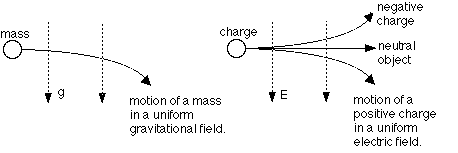### What does an electric field look like?

An electric field can be visualized on paper by drawing lines of force, which give an indication of both the size and the strength of the field. Lines of force are also called field lines. Field lines start on positive charges and end on negative charges, and the direction of the field line at a point tells you what direction the force experienced by a charge will be if the charge is placed at that point. If the charge is positive, it will experience a force in the same direction as the field; if it is negative the force will be opposite to the field.

The fields from isolated, individual charges look like this: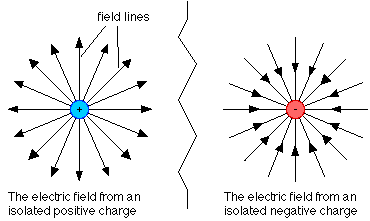When there is more than one charge in a region, the electric field lines will not be straight lines; they will curve in response to the different charges. In every case, though, the field is highest where the field lines are close together, and decreases as the lines get further apart.

### An example

Two charges are placed on the x axis. The first, with a charge of +Q, is at the origin. The second, with a charge of -2Q, is at x = 1.00 m. Where on the x axis is the electric field equal to zero?This question involves an important concept that we haven't discussed yet: the field from a collection of charges is simply the vector sum of the fields from the individual charges. To find the places where the field is zero, simply add the field from the first charge to that of the second charge and see where they cancel each other out.

In any problem like this it's helpful to come up with a rough estimate of where the point, or points, where the field is zero is/are. There is no such point between the two charges, because between them the field from the +Q charge points to the right and so does the field from the -2Q charge. To the right of the -2Q charge, the field from the +Q charge points right and the one from the -2Q charge points left. The field from the -2Q charge is always larger, though, because the charge is bigger and closer, so the fields can't cancel. To the left of the +Q charge, though, the fields can cancel. Let's say the point where they cancel is a distance x to the left of the +Q charge.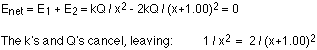Cross-multiplying and expanding the bracket gives:Solving for x using the quadratic equation gives: x = 2.41 m or x = -0.414 m

The answer to go with is x = 2.41 m. This corresponds to 2.41 m to the left of the +Q charge. The other point is between the charges. It corresponds to the point where the fields from the two charges have the same magnitude, but they both point in the same direction there so they don't cancel out.

### The field around a charged conductor

A conductor is in electrostatic equilibrium when the charge distribution (the way the charge is distributed over the conductor) is fixed. Basically, when you charge a conductor the charge spreads itself out. At equilibrium, the charge and electric field follow these guidelines:

• the excess charge lies only at the surface of the conductor
• the electric field is zero within the solid part of the conductor
• the electric field at the surface of the conductor is perpendicular to the surface
• charge accumulates, and the field is strongest, on pointy parts of the conductor

Let's see if we can explain these things. Consider a negatively-charged conductor; in other words, a conductor with an excess of electrons. The excess electrons repel each other, so they want to get as far away from each other as possible. To do this they move to the surface of the conductor. They also distribute themselves so the electric field inside the conductor is zero. If the field wasn't zero, any electrons that are free to move would. There are plenty of free electrons inside the conductor (they're the ones that are canceling out the positive charge from all the protons) and they don't move, so the field must be zero.

A similar argument explains why the field at the surface of the conductor is perpendicular to the surface. If it wasn't, there would be a component of the field along the surface. A charge experiencing that field would move along the surface in response to that field, which is inconsistent with the conductor being in equilibrium.

Why does charge pile up at the pointy ends of a conductor? Consider two conductors, one in the shape of a circle and one in the shape of a line. Charges are distributed uniformly along both conductors. With the circular shape, each charge has no net force on it, because there is the same amount of charge on either side of it and it is uniformly distributed. The circular conductor is in equilibrium, as far as its charge distribution is concerned.With the line, on the other hand, a uniform distribution does not correspond to equilbrium. If you look at the second charge from the left on the line, for example, there is just one charge to its left and several on the right. This charge would experience a force to the left, pushing it down towards the end. For charge distributed along a line, the equilibrium distribution would look more like this: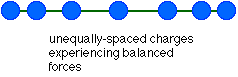The charge accumulates at the pointy ends because that balances the forces on each charge.

### Electric flux

A clever way to calculate the electric field from a charged conductor is to use Gauss' Law, which is explained in Appendix D in the textbook. Gauss' Law can be tricky to apply, though, so we won't get into that. What we will do is to look at some implications of Gauss' Law. It's also a good time to introduce the concept of flux. This is important for deriving electric fields with Gauss' Law, which you will NOT be responsible for; where it'll really help us out is when we get to magnetism, when we do magnetic flux.

Electric flux is a measure of the number of electric field lines passing through an area. To calculate the flux through a particular surface, multiply the surface area by the component of the electric field perpendicular to the surface. If the electric field is parallel to the surface, no field lines pass through the surface and the flux will be zero. The maximum flux occurs when the field is perpendicular to the surface.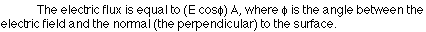### Permittivity

Even though we won't use this for anything, we should at least write down Gauss' law:

Gauss' Law - the sum of the electric flux through a surface is equal to the charge enclosed by a surface divided by a constant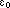, the permittivity of free space.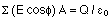What is the permittivity of free space? It's a constant related to the constant k that appears in Coulomb's law. The relationship between the two is this: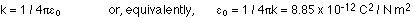### Implications of Gauss' Law

Gauss' Law is a powerful method of calculating electric fields. If you have a solid conducting sphere (e.g., a metal ball) that has a net charge Q on it, you know all the excess charge lies on the outside of the sphere. Gauss' law tells us that the electric field inside the sphere is zero, and the electric field outside the sphere is the same as the field from a point charge with a net charge of Q. That's a pretty neat result.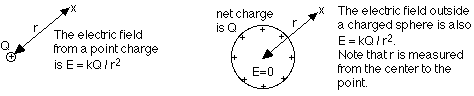The result for the sphere applies whether it's solid or hollow. Let's look at the hollow sphere, and make it more interesting by adding a point charge at the center.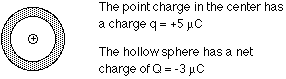What does the electric field look like around this charge inside the hollow sphere? How is the negative charge distributed on the hollow sphere? To find the answers, keep these things in mind:

• The electric field must be zero inside the solid part of the sphere
• Outside the solid part of the sphere, you can find the net electric field by adding, as vectors, the electric field from the point charge alone and from the sphere alone

We know that the electric field from the point charge is given by kq / r2. Because the charge is positive, the field points away from the charge.

If we took the point charge out of the sphere, the field from the negative charge on the sphere would be zero inside the sphere, and given by kQ / r2 outside the sphere.

The net electric field with the point charge and the charged sphere, then, is the sum of the fields from the point charge alone and from the sphere alone (except inside the solid part of the sphere, where the field must be zero). This is shown in the picture: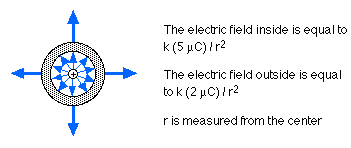How is the charge distributed on the sphere? The electrons must distribute themselves so the field is zero in the solid part. This means there must be -5 microcoulombs of charge on the inner surface, to stop all the field lines from the +5 microcoulomb point charge. There must then be +2 microcoulombs of charge on the outer surface of the sphere, to give a net charge of -5+2 = -3 microcoulombs.